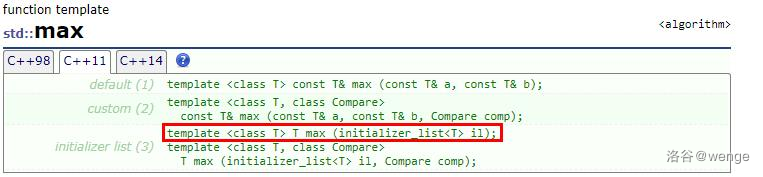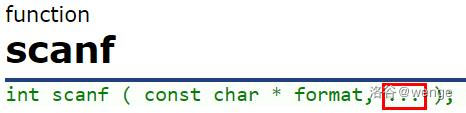2019-09-05 21:36:57

• 子函数的编写（必需）

• C++的类型

• 强制类型转换

• 指针/迭代器

• auto

• 模板

## 2.引言

ans=max(a,b);

wenge又问你：

ans=max(a,max(b,c));

wenge又问你：

ans=max(a,max(a,max(a,max(a,max(a,max(a,max(a,max(a,max(a,a)))))))));

wenge说这太乱了。

ans=max(ans,a);
ans=max(ans,a);
ans=max(ans,a);
ans=max(ans,a);
ans=max(ans,a);
ans=max(ans,a);
ans=max(ans,a);
ans=max(ans,a);
ans=max(ans,a);
ans=max(ans,a);

ans=max({a,a,a,a,a,a,a,a,a,a});//C++11

## 3.C++ STL的可变参数（initializer_list）

### 1）什么是initializer_list{1,2,3,4,5}

vector<int> a={1,2,3,4,5};

int a={1,2,3,4,5};

initializer_list是C++11的一个新特性。这玩意除了能给数组赋值以外，还可以给自己定义的结构体赋值。比如

#include <iostream>
#include <initializer_list>
struct point{
int x,y;
};

int main(){
point a={1,2};
cout<<a.x<<" "<<a.y;
return 0;
}

### 2）initializer_list实现可变参数

initializer_list实现可变参数的方式，就是把initializer_list作为函数的参数。

#include <iostream>
#include <algorithm>
#include <initializer_list>
using namespace std;
//C++ STL的可变参数

int mymax(initializer_list<int> a){
int ans=-2147483648;//int的最小值
for(auto i:a){
ans=max(i,ans);
}
return ans;
}

int main(){
int a=1,b=2,c=3,d=4,e=5;
cout<<mymax({a,b,c,d,e});
return 0;
}

int mymax(initializer_list<int> a){
int ans=-2147483648;//int的最小值
for(initializer_list<int>::iterator i=a.begin();i!=a.end();i++){
ans=max(*i,ans);
}
return ans;
}

#include <iostream>
#include <algorithm>
#include <initializer_list>
using namespace std;

int b;

int average(initializer_list<int> a){
int ans=0;
int j=1;
for(auto i:a){
b[j]=i;
j++;
}
sort(b+1,b+a.size()+1);
for(int i=2;i<a.size();i++){
ans+=b[i];
}
ans/=(a.size()-2);
return ans;
}
struct point{
int x,y;
};

int main(){
int a=80,b=10,c=40,d=40,e=70;
cout<<average({a,b,c,d,e});
return 0;
}

void print(initializer_list<int> a,initializer_list<char> b);//print()的具体定义略
int main(){
print({1,2,3},{'a','b','c'});
return 0;
}

template<typename T>
void print(initializer_list<T> a);//print()的具体定义略
int main(){
print({114514,1919,810});
print({'c','h','a','r'});
return 0;
}

### 3）类型转换问题以及其他需要注意的地方

C++标准要求“initializer_list的元素类型都必须相同，但编译器将进行必要的转换”、“但不能进行隐式的窄化转换”（C++ Primer）。比如下面的代码：

double a={1.14514,2.33,-3.456789,4.0,5};
//int类型的5被编译器隐式转换成double类型的5.0

long long b={114514ll,233ll,-3456789ll,4ll,5};
//int类型的5被编译器隐式转换成long long类型的5

int c={114514,233,-3456789,4,5.0};
//double类型的5.0被编译器转换成int，属于隐式窄化转换，错误 

void print(initializer_list<int> a);//print()的具体定义略
int main(){
print("string");
return 0;
}

## 4.C的可变参数（va_list）

### 1）从scanf和printf说起va_list

### 2）va_list实现可变参数

C/C++函数可以通过在其普通参数后添加逗号和三个点（,...）的方式来接受数量不定的附加参数，而无需相应的参数声明。

void print(int count,...);//合法
void print(...);//合法

void print(int count,...,int count2);//非法，CE

C/C++头文件<stdarg.h><cstdarg>提供了对可变参数的支持。va_list是一个类型，定义在头文件<stdarg.h><cstdarg>中。<stdarg.h>中定义了3个宏，与va_list配套使用，分别是va_listva_startva_argva_end。在C++中，C++11标准又新增了宏va_copy。所以，va_listva_startva_argva_end是C与C++通用的。

#include <iostream>
#include <cstdarg>
using namespace std;

void printint(int count,...){
va_list a;
va_start(a,count);
for(int i=1;i<=count;i++){
int b=va_arg(a,int);
cout<<b<<" ";
}
va_end(a);
}
int main(){
printint(5,114514,233,-3456789,4,5);
return 0;
}

1. 在函数体内，我们首先定义了一个va_list类型的aa即是printint()的参数表，即其包含了包括count在内的所有参数。你可以把va_list看做一个栈（实际上也是这么存储的），参数从右至左入栈。

2. 然后，我们调用了va_start宏。va_start宏有两个参数，第一个需要写我们之前创建的va_list的名字（在这个例子里是a），第二个参数则写...之前的上一个参数（在这个例子里是count）。你可以想象有一个指针，一开始什么都不指向（即指向NULL），我们调用va_start宏，则这个指针就指向了栈顶，并且一直弹栈，直到指针指向了...之前的上一个参数（在这个例子里是count）的位置。

3. 然后，我们使用va_arg宏读取函数的参数。va_arg宏有两个参数，第一个需要写我们之前创建的va_list的名字，第二个填写当前所要读取的参数的类型（这也是scanf为什么有那么多占位符的原因，因为它必须获取参数的类型）。你可以认为这个宏先进行弹栈，然后读取栈顶内容并返回。注意，va_arg的第二个参数中，char,char_16t,wchar_t,short以及其signed,unsigned版本要写成intfloat要写成doublechar_32t要写成unsigned long。原因是C/C++的默认类型转换。否则必定RE。这个东西gcc会给出warning。除此之外，如果第二个参数的实际类型不同于va_arg的第二个参数，这个参数会被吃掉。

4. 最后，使用va_end宏结束函数参数的读取。你可以认为这个宏使指针重新指向NULL，并且删除赋予va_list的内存，使可变参数函数能正确返回。有助于代码的健壮。

#include <iostream>
#include <cstdarg>
using namespace std;

void printint(int first,...){
va_list a;
int b=first;
va_start(a,first);
while(b!=-1){
cout<<b<<" ";
b=va_arg(a,int);
}
va_end(a);
}
int main(){
printint(114514,233,-3456789,4,5,-1);
return 0;
}

#include <iostream>
#include <cstdarg>
using namespace std;

void myprintf(string f,...){
va_list a;
va_start(a,f);
for(int i=0;i<f.size();i++){
if(f[i]=='%'){
i++;
if(f[i]=='d'){;
cout<<va_arg(a,int);
}
if(f[i]=='c'){
cout<<char(va_arg(a,int));
}
}
else cout<<f[i];
}
va_end(a);
}
int main(){
myprintf("test\n%d%c",114514,'A');
return 0;
}

#include <iostream>
#include <cstdarg>
using namespace std;

void myprintf(string f,...){
va_list a,b;
va_copy(b,a);
va_start(a,f);
for(int i=0;i<f.size();i++){
if(f[i]=='%'){
i++;
if(f[i]=='d'){;
cout<<va_arg(a,int);
}
if(f[i]=='c'){
cout<<char(va_arg(a,int));
}
}
else cout<<f[i];
}
va_end(a);
va_start(b,f);
for(int i=0;i<f.size();i++){
if(f[i]=='%'){
i++;
if(f[i]=='d'){;
cout<<va_arg(b,int);
}
if(f[i]=='c'){
cout<<char(va_arg(b,int));
}
}
else cout<<f[i];
}
va_end(b);
}
int main(){
myprintf("test\n%d%c",114514,'A');
return 0;
}


### 3）其他

#include <iostream>
#include <cstdarg>
using namespace std;

void myprintf(string f,...){
va_list a;
va_start(a,f);
for(int i=0;i<f.size();i++){
if(f[i]=='%'){
i++;
if(f[i]=='d'){;
cout<<va_arg(a,int);
}
if(f[i]=='c'){
cout<<va_arg(a,int);
//没有转换，正确写法实际上是用强制类型转换
//cout<<char(va_arg(a,int));
}
}
else cout<<f[i];
}
va_end(a);
}
int main(){
myprintf("%c",'A');
return 0;
}


## 5.参考资料

http://www.cplusplus.com/

C++ Primer

va_start和va_end使用详解

• star
首页